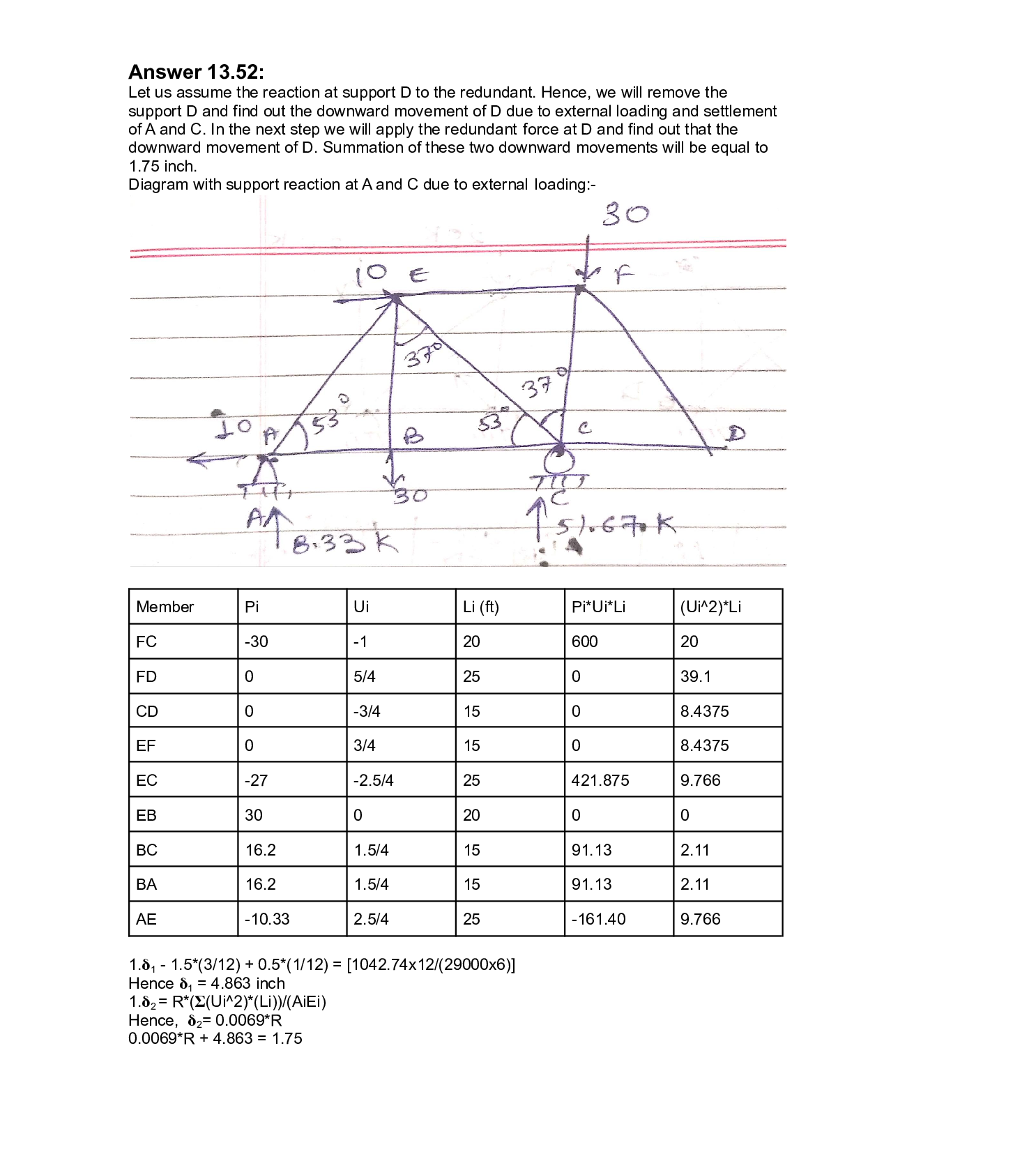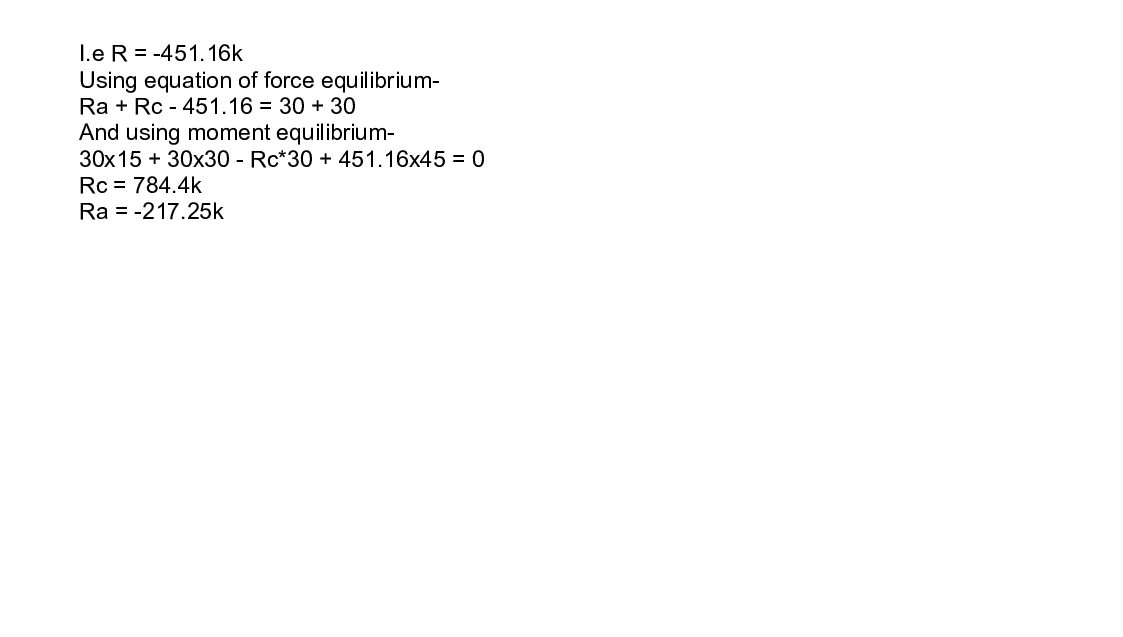Question

Structural Analysis

13.52 Solve Problem 13.27 for the loading shown in Fig.P13.27 and the support settlements of 1 in. at 4, 3 in. at C,and 1 in. at D.Verified### Question 45402Structural Analysis

5) For the truss given below, find the force in member EF. Assume that the self-weight ofthe truss is negligible. (15 pts)

### Question 45401Structural Analysis

4) From the options given below, choose the most economical size HSS brace that will beable to resist the force shown. Assume that the brace will be designed as a tension-onlybrace (no compression). (10 points)

### Question 45400Structural Analysis

3) For the beam given below, calculate reactions at both supports A and B. Draw the shear and moment diagrams. Label the maximum magnitude shear and moment values on the diagrams. (Maximum magnitude means maximum absolute value, positive or negative).Assume that the self-weight of the beam is negligible. (20 pts)

### Question 45399Structural Analysis

1) Consider the two columns given below. Both have the same material properties. Both have equal unbraced lengths about their respective x and y axes. Both have the same end conditions (pin-pin). However, one is square in shape, and the other is rectangular.Which column is more efficient? Justify your answer by comparing the critical buckling load of each column and the amount of material used. (15 pts)
2) For the beam given below, calculate the vertical reaction at support A. Assume that the-self-weight of the beam is negligible. (15 pts)
3) For the beam given below, calculate reactions at both supports A and B. Draw the shearand moment diagrams. Label the maximum magnitude shear and moment values on thediagrams. (Maximum magnitude means maximum absolute value, positive or negative).Assume that the self-weight of the beam is negligible. (20 pts)
4) From the options given below, choose the most economical size HSS brace that will be able to resist the force shown. Assume that the brace will be designed as a tension-only brace (no compression). (10 points)
5) For the truss given below, find the force in member EF. Assume that the self-weight of the truss is negligible. (15 pts)

### Question 45398Structural Analysis

1) In addition to recording the size, section and relative locations of structural members(design output), IBC 1603.1 also states that structural engineers must indicate______(design input) on the- (4 pts)
2) A flexible building will have ato a rigid building. The flexible building will experiencecompared to the more rigid building. (3 pts)frequency and aperiod comparedseismic forces
3) The IBC lists site classifications for types of soil, from hard rock to soft soil prone toliquefaction (A - F). Typically, theengineer will perform tests onthe soil to determine the site classification. Structures built onsoilwill experience higher seismic accelerations due to wave(3 pts)
4) Carbon dioxide emitted during the manufacturing, transportation, and construction of building materials (including structural elements) is known a______carbon. (2 pts)
5) You are designing a truss for a sports arena. The owner would like to hang a 20 kipscoreboard in the center of one of these trusses. They have proposed attaching thecables at the locations shown below. What change, if any, would you propose to improvethe efficiency of the truss? Justify your answer. (4 pts)
6) The metal clips in the wall at the top of the photo (A) will support the brick veneer againstloads. The shelf at the floor level (B) will support theof the brick. (4 pts)
7) You are testing the tensile capacity of two rods made of different metals. The first rod that you test elongates rapidly and ruptures after applying 2,000 pounds of load. The second rod hardly elongates at all and ruptures after applying 4,000 pounds. On thestress-strain curve below, the first rod is_______The second rod is____---The rod with the higher ductility is- (3 pts)
8) In structural analysis, the type of support or boundary condition circled below is commonly referred to as a_{___(2 pts)

### Question 44751Structural Analysis

3. (20 points) Find the most economic beam section for the following loading condition based on flexure ONLY. Note that there is a bracing at the center of the span. Neglect beam's self-weight.

### Question 44750Structural Analysis

(15 points) In the simply supported beam AB below, please find the maximum moment,maximum shear and maximum deflection and indicate where they occur.

### Question 44749Structural Analysis

What is the volume of (42+x =) ft. long W 33 x 318 steel section?

### Question 44620Structural Analysis

A structural engineer has hired you to design square concrete unembedded footings for a building, based only on capacity. Each footing will hold an axial load of 340 kN. Your technicians have conducted lab tests on specimens sampled from the site, and they report the following profile: a 0.8m thick clay layer over a 7m thick clayey silt layer (the clayey silt layer lies over rock). The lab report reveals that the clay has a friction angle of34 degrees and an undrained strength of 35 kPa, and that the clayey silt has a friction angle of 37 degrees and an undrained strength of 45 kPa.
Determine the size of one of the footings, in meters, such that the critical actor of safety against a capacity failure is as close to 3 as possible, but still larger than 3 and smaller than 3.2. The other (non-critical) factor of safety must be greater than 3. Express your answer with a resolution of 0.1 meters. That is, values of B of1.2, 2.3, 3.4m, etc., for example, are acceptable, whereas B values of 1.21, 2.32, 3.43m, etc., for example,are not.

### Question 44411Structural Analysis

2. (50 points) Determine if the beam shown in the figure is adequate as governed by the ACICode (318-11). The loads shown are service loads. The uniformly distributed load is DL = 0..72+o.Fkip/ft., LL+0.83 +0.E= kip/ft the dead load excludes the beam weight the point is a live load f.c 4000psi fy=60000 psi

### Submit query